Courses

# Matrix MCQ - 2

## 30 Questions MCQ Test Topic-wise Tests & Solved Examples for IIT JAM Mathematics | Matrix MCQ - 2

Description
This mock test of Matrix MCQ - 2 for Mathematics helps you for every Mathematics entrance exam. This contains 30 Multiple Choice Questions for Mathematics Matrix MCQ - 2 (mcq) to study with solutions a complete question bank. The solved questions answers in this Matrix MCQ - 2 quiz give you a good mix of easy questions and tough questions. Mathematics students definitely take this Matrix MCQ - 2 exercise for a better result in the exam. You can find other Matrix MCQ - 2 extra questions, long questions & short questions for Mathematics on EduRev as well by searching above.
QUESTION: 1

Solution:
QUESTION: 2

Solution:
QUESTION: 3

###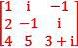is a 3 X 3 matrix over the set of

Solution:
QUESTION: 4

If A =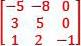,  then A2 is

Solution:
QUESTION: 5

1 x n matrices are called

Solution:
QUESTION: 6

Let f(x) = x2 — 5x + 6 and A =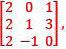then f (A) is equal

Solution:
QUESTION: 7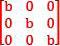is called a

Solution:
QUESTION: 8

X = [X1, X2, ... , Xn] is an n — tuple non — zero vector. Then n x n matrix V = VX

Solution:
QUESTION: 9

A submatrix of the given matrix can be obtained by deleting

Solution:
QUESTION: 10

Consider a non — homogeneous system of linear equation represented mathematically an over determined system. Such a system will be

Solution:
QUESTION: 11

Two matrices A and B are said to be comparable if

Solution:
QUESTION: 12

Consider the system of equation A(nxn)X(nx1) = λ nx1 where, λ is a scalar. Let, (λi, xi) be an eigen pair of an eigen value and its corresponding eigen vector for real matrix A. Let I be a (n x n) unit matrix. Which one of the following statements is not correct

Solution:
QUESTION: 13

If A =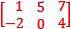and B =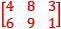then A x B =

Solution:
QUESTION: 14

Eigen values of a matrix S =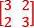are 5 and 1. What is the eigen values of the matrix S2 = SS?

Solution:
QUESTION: 15

If A is non - scalar, non - identity idempotent matrix of order n ≥ 2. Then, minimal polynomial mA(x) is

Solution:
QUESTION: 16

If 4X =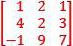then X

Solution:
QUESTION: 17

If A is a non scalar non identity idempotent matrix of order n≥2. the minimal polynomial mA(x) is

Solution:

Since A is idempotent A2 = A

A2 - A = 0

mA(x) = x2 - x

= x(x-1)

QUESTION: 18

If A =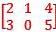and B =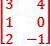then AB is a matrix of order

Solution:
QUESTION: 19

All the four entries of the 2 x 2 matrix P =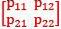are non - zero, and one of its eigen values is zero . Which one of the following statements is true?

Solution:
QUESTION: 20

In the matrix equation PX = q, which of the following is necessary condition for the existence of atleast one solution for the unknown vector

Solution:
QUESTION: 21

If D1, Dare two diagonal matrices, then

Solution:
QUESTION: 22

Matrix multiplication is

Solution:
QUESTION: 23

The rank of the following (n + 1 ) x (n + 1) matrix where a is the real number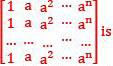Solution: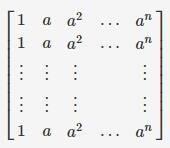R2→R2−R1,R3→R3−R1,R4→R4−R1, and so on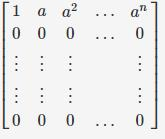Rank of matrix = 1

QUESTION: 24

Multiplication of matrices E and F is G. Matrices E and G are as follows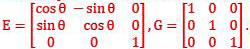Then, the value of matrix F is

Solution:
QUESTION: 25

The linear operator L(x) is defined by the cross product L(x) = b x X, where b = [0 1 0]T and X = [x1 x2 x3]T are three dimensional vectors. The 3 x 3 matrix M o f this operation satisfies L (x ) =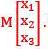Then, the eigen values of M are

Solution:
QUESTION: 26

Which one of the following is not an elementary operation?

Solution:
QUESTION: 27

Which one of the following statements is incorrect for an upper triangular matrix A = (aij)?

Solution:
QUESTION: 28

Mark the correct definition of rank of a matrix

Solution:
QUESTION: 29

The rank of matrix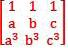a, b, c, being all real, is 3 if

Solution:
QUESTION: 30

Two matrics Aand B are said to anti — commute if

Solution: